Algebra 1 4-6 Complete Lesson: Formalizing Relations and Functions
starstarstarstarstarstarstarstarstarstar
4.5 (1 rating)
by Matthew Richardson
| 31 Questions
Note from the author:
A complete formative lesson with embedded slideshow, mini lecture screencasts, checks for understanding, practice items, mixed review, and reflection. I create these assignments to supplement each lesson of Pearson's Common Core Edition Algebra 1, Algebra 2, and Geometry courses. See also mathquest.net and twitter.com/mathquestEDU.The outlined content above was added from outside of Formative.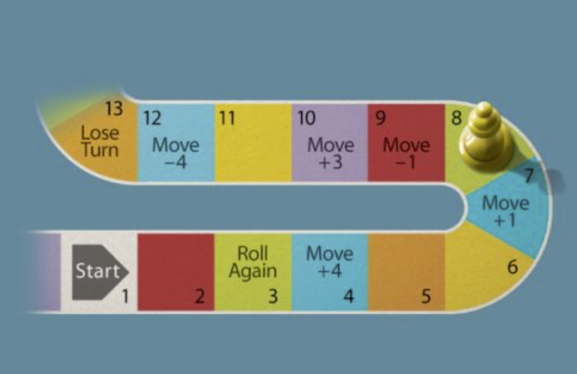1
1
10 pts
Solve It! Your friend is playing a board game. He is on the space shown in the diagram. He rolled a 3 to get to that space. On which spaces could he have started before rolling the 3? Select all that apply.
1
2
3
4
5
6
7
8
9
102
10 pts
Problem 1 Got It? Identify the domain and range of the relation.
Place each value only once.
• 4.2
• 1.5
• 5
• 2.2
• 7
• 4.8
• 0
• Domain
• Range
3
10 pts
Problem 1 Got It? Represent the relation with a mapping diagram.
4
5 pts
Problem 1 Got It? Is the relation a function?

Yes
No
5
10 pts
Problem 1 Got It? Identify the domain and range of the relation.
Place each value only once.
• -1
• 1
• -2
• 2
• 4
• -4
• 7
• -7
• Domain
• Range
6
10 pts
Problem 1 Got It? Represent the relation with a mapping diagram.
7
5 pts
Problem 1 Got It? Is the relation a function?

Yes
No8
8
5 pts
Problem 2 Got It?
A
B9
9
5 pts
Problem 2 Got It?
A
B10
10
10 pts
Problem 3 Got It? Use the function in Problem 3, w(x) = 250x. How many words can you read in 6 min? Enter only a number.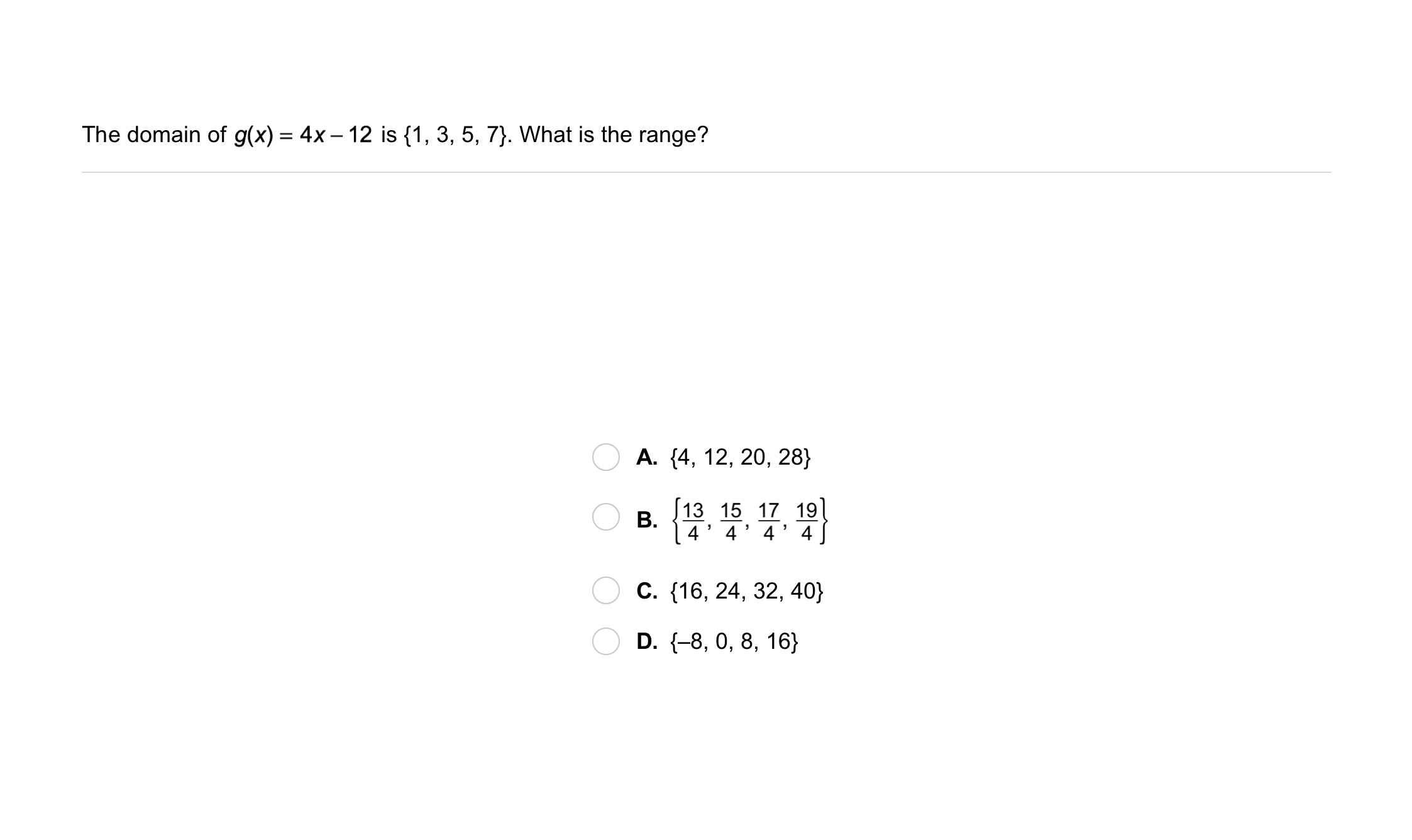11
11
10 pts
Problem 4 Got It?
A
B
C
D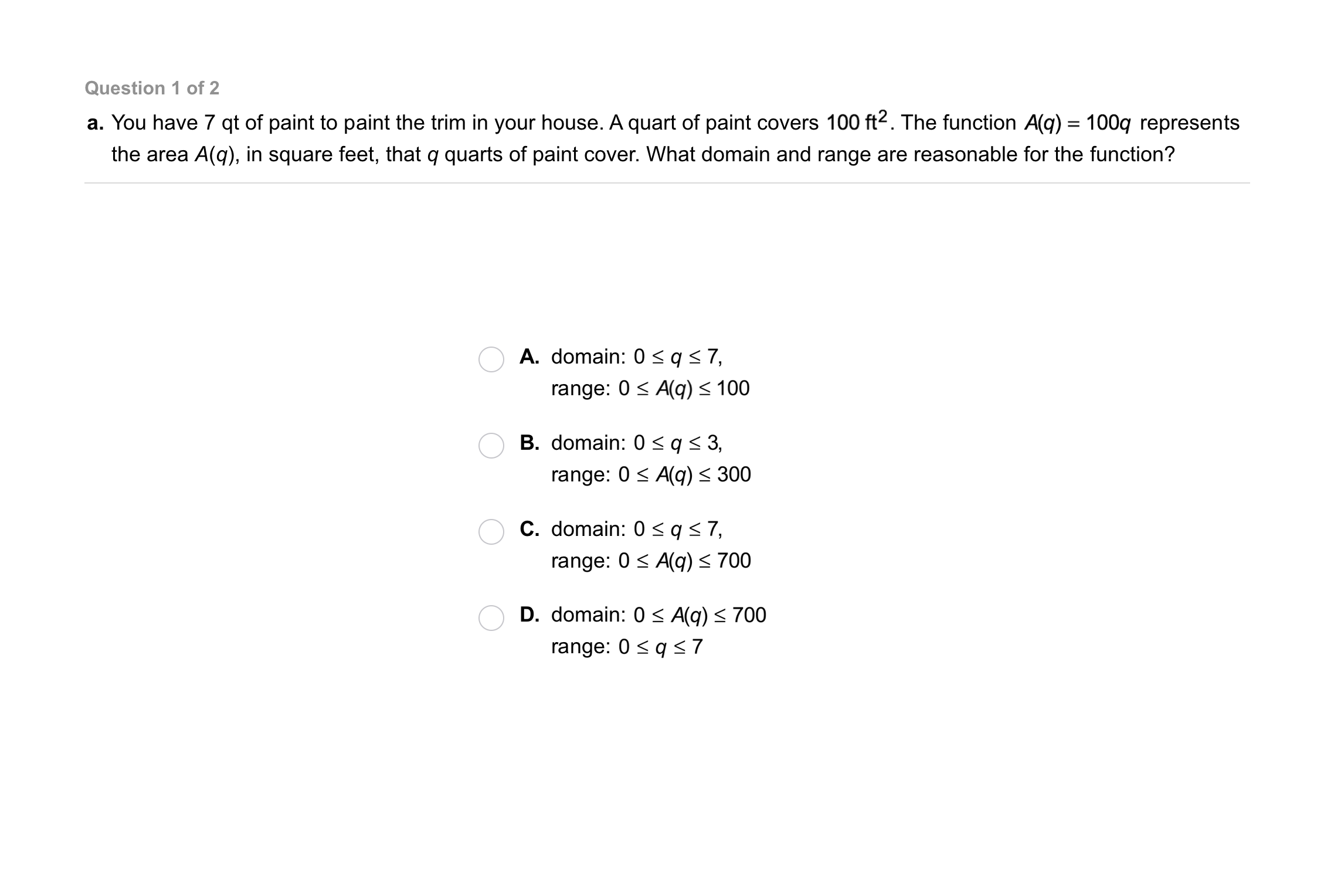12
12
10 pts
Problem 5 Got It?
A
B
C
D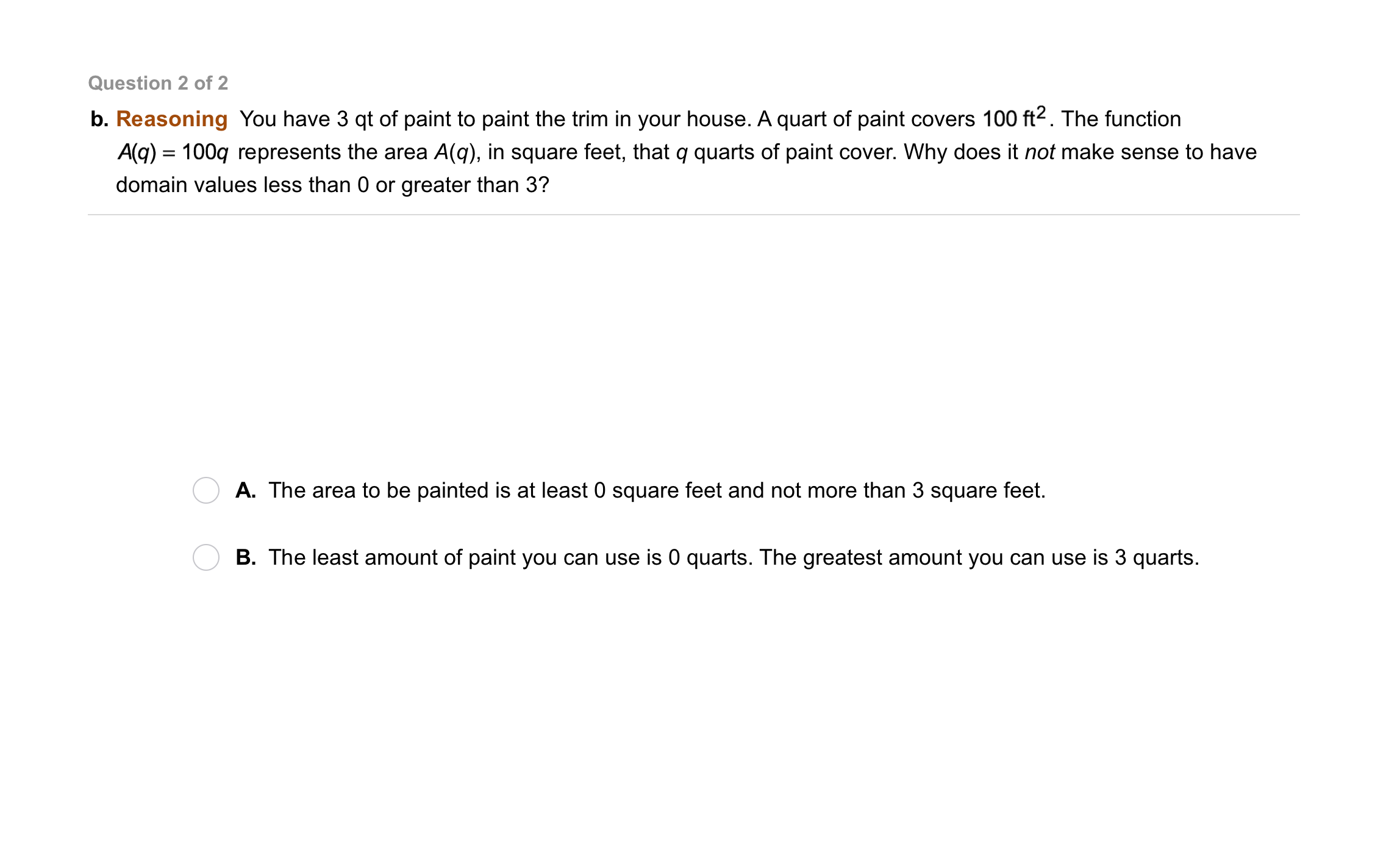13
13
5 pts
Problem 5 Got It?
A
B14
10 pts
Consider the relation {(-2, -3), (-1, 4), (0, 5), (1, 6)}.

Identify the domain of the relation.
{-3, -2, -1, 0, 1, 5, 6}
{-2, -1, 0, 1}
{-3, 4, 5, 6}
15
10 pts
Consider the relation {(-2, -3), (-1, 4), (0, 5), (1, 6)}.

Identify the range of the relation.
{-2, -1, 0, 1}
{-3, -2, -1, 0, 1, 5, 6}
{-3, 4, 5, 6}
16
10 pts
Consider the relation {(-2, -3), (-1, 4), (0, 5), (1, 6)}.

Represent the relation with a mapping diagram. Remember to label the domain and range in your diagram.17
17
5 pts
Is the relation in the graph a function? Use the vertical line test.
No, it is not a function.
Yes, it is a function.
18
10 pts
What is f(2) for the function f(x) = 4x + 1?
-2
3
9
43
19
10 pts
The domain of f(x), defined below, is {-4, -2, 0, 2, 4}. What is the range?

{-8, -4, 0, 4, 8}
{-2, -1, 0, 1, 2}
{-2, -1, 1, 2}20
5 pts
Vocabulary: Write y = 2x + 7 using function notation.
f(x) = 2x + 7
f(x) = {y|y is a Real number, y = 2x + 7}
21
20 pts
Compare and Contrast: You can use a mapping diagram or the vertical line test to tell if a relation is a function. Which method do you prefer? Explain.22
22
10 pts
Error Analysis: A student drew the dashed line on the graph shown and concluded that the graph represented a function. Is the student correct? Explain.23
10 pts
Review Lesson 4-5: Match each function rule with the situation it models.
• E = 7 + 5h
• a = 4.5s + 10
• You buy several pairs of socks for \$4.50 per pair, plus a shirt for \$10. The total amount a you spend is a function of the number of pairs of socks you buy.
• You babysit for \$5 per hour and get a \$7 tip. Your earnings E are a function of the number of hours h you work.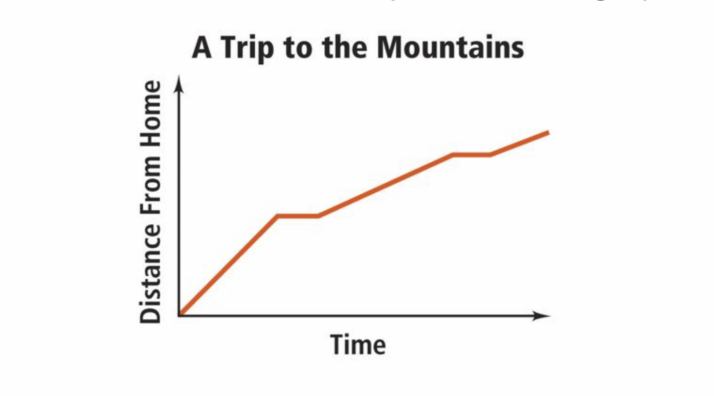24
24
10 pts
Review Lesson 4-1: The graph shows a family's distance from home as they drive to the mountains for a vacation. Label the variables in the graph.
• variable
• Number of trips
• Time
• Elevation
• Number of deer spotted
• Distance from home
25
30 pts
Review Lesson 4-1: Describe how the variables are related at the indicated points on the graph.
26
10 pts
Review Lesson 1-2: Evaluate the expression for x = 1, 2, 3, and 4.
• x = 1
• x = 2
• x = 3
• x = 4
• Expression = 18
• Expression = 12
• Expression = 9
• Expression = 15
27
10 pts
Review Lesson 1-2: Evaluate the expression for x = 1, 2, 3, and 4. Only use each x-value once.
Not all expression value categories will be used.
• x = 1
• x = 2
• x = 3
• x = 4
• Expression = 7
• Expression = 15
• Expression = 29
• Expression = 22
• Expression = 828
10 pts
Vocabulary Review: Use the words in the left column to label the function machine in the image below. Use each word once.

• function rule
• y-values
• output
• x-values
• input
• range
• Region A
• Region B
• Region C29
10 pts
Use Your Vocabulary: Complete each sentence with the appropriate word from the column on the left. Use each word once.
• reasonable
• reasonableness
• unreasonable
• The student estimated to check the __?__ of her answer.
• Sales tax of \$32 on an \$85 item is __?__.
• A price of \$14 is __?__ for a pizza.
30
100 pts
Notes: Take a clear picture or screenshot of your Cornell notes for this lesson. Upload it to the canvas. Zoom and pan as needed.